• 本节开始介绍第一个生成模型朴素贝叶斯模型(NB model)。生成模型与判别式模型的区别在于，生成模型对样本的联合分布建模: 　 因此我们需要求出样本的类别分布p(y)与，样本类概率分布p(x|y)。生成模型的...
　　本节开始介绍第一个生成式模型：朴素贝叶斯模型(NB model)。生成式模型与判别式模型的区别在于，生成式模型对样本的联合分布建模:因此我们需要求出样本的类别分布p(y)与，样本类概率分布p(x|y)。生成式模型的困难在于p(x|y)计算，有时我们很难去估计类概率分布。因此，我们会做出一些假设，Naive Bayes Assumption 是指：样本在给定类别的条件下，各个特征之间相互独立：根据p(xi|y)分布的假设不同，我们可以分成两种假设模型：muti-variate Bernouli model 与 multinomial event model，以下将分两个部分介绍这两种模型。
1.muti-variate Bernouli model
这种模型对应于文本分类中，特征权重是BOOL型表示方法，仅关心文本中是否出现某个词。假设当前样本集的字典V=[v1,v2,v3...v|k|]，字典的每一个词对应一次Bernouli实验。对于某个样本x=[t1,t2,t3...t|v|]，其类概率密度：记Θi|j=p(ti|cj),Θj=p(y=cj)。因此样本的联合概率分布可以写成：对于训练集{(xk,yk)}(k=1,2,3,...m)，其最大似然估计：其中满足条件:采用拉格朗日乘子法，可以求得：2.Mutinomianl event model
多项式模型对应文本中的词频特征权重（Term Frequency），不仅关系某个词是否出现，而且还关心起在文档里出现的次数。假设一篇文档x=[w1,w2,...w|h|,...w|x|]其中w|h|表示文档x第h个单词，在贝叶斯假设下类概率密度函数：使用词典模型表示该篇文档x=[t1,t2,...t|v|]，其中ti 表示字典第i个词在文档中出现的次数，因此上式可以改下成：因此，样本的联合概率分布：对于样本集：D={(xk,yk)}  (k=1,2...N)，最大似然估计：其中满足条件：采用拉格朗日乘子法：ps:老板写的Naive Bayes开源工具，上面的推导也是老板的一个课件内容整理。
地址：http://www.openpr.org.cn/index.php/NLP-Toolkit-for-Natural-Language-Processing/43-Naive-Bayes-Classfier/View-details.html

转载于:https://www.cnblogs.com/wangbogong/archive/2013/05/08/3067162.html
展开全文• ## 朴素贝叶斯

千次阅读 2014-04-14 09:08:58
朴素贝叶斯事件模型 2、 神经网络（简要） 3、 支撑向量机（SVM）铺垫 – 最大间隔分类器   复习： 1、 朴素贝叶斯 一种生成学习算法，对p(x|y)建模。   例：垃圾邮件分类 以...

朴素贝叶斯

本次课程大纲：

1、 朴素贝叶斯

-          朴素贝叶斯事件模型

2、 神经网络（简要）

3、 支撑向量机（SVM）铺垫 – 最大间隔分类器

复习：

1、 朴素贝叶斯

一种生成学习算法，对p(x|y)建模。

例：垃圾邮件分类

以邮件输入流作为输入，输出y为{0,1}，1为垃圾邮件，0为非垃圾邮件。

将邮件文本表示为一个输入向量x

1）  xi∈{0,1}，表示字典中的第i个词是否出现在邮件中

2）  x长度为n，n为字典的词数

3）  该模型称为多元伯努利事件模型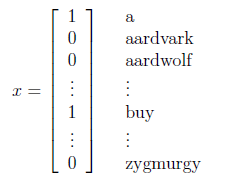假设xi在给定y的时候是条件独立的，则x在给定y下的概率可简化为：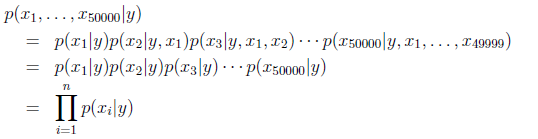根据朴素贝叶斯公式，求p(y|x)最大时的y：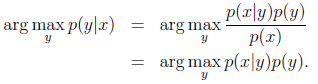算法变化版本：

1）  让xi取多个值，xi∈{1,2,…,k}，类似上式有：p(x|y) = ∏ p(xi|y)，但是p(xi|y)变成多项式分布，而不是伯努利分布。

例：估计房屋面积预测房屋能否被卖掉，将房屋面积分成几个离散区间，如0-,1000为xi=1,1000-1500为xi=2，1500-2000为xi=3,2000以上为xi=4

2）  如上例处理邮件（文本）中，x向量记录每个词出现的次数（而不是是否出现）

多项式事件模型

接上例，给出一封邮件，将它表示成特征向量：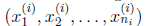，ni表示邮件中词的数量，xj是个到词典的索引，表示该词在词典的位置。

如邮件中有300个词，那么特征向量x(i)长度为300，若词典有50000个词，每个元素xj的取值范围为{1,2,…,50000}

则生成模型的联合概率p(xy)为：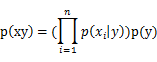n为邮件长度

上式理解：邮件内容满足一些概率分布，有一些随机分布在生成这些邮件。过程为：首先确定y，即是否为垃圾邮件，决定一个人是否向你发送垃圾邮件后，遍历邮件的300个词，按照某种概率分布生成一些词，基于他们是否向你发送垃圾邮件

模型参数：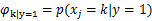表示某人决定向你发送垃圾邮件(y=1)时，选择词k的概率，类似有：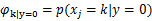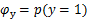给出训练集后，求极大似然估计：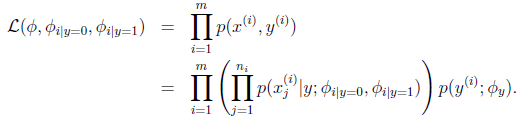得到：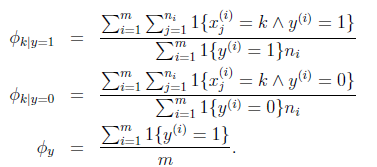上面第一个式子，分子的意思是，对所有标签为1的邮件求和，之后对垃圾邮件中的词k求和，所以分子实际上就是训练集中所有垃圾邮件中词k出现的次数。分母是训练集中所有垃圾邮件的长度。比值的含义就是所有垃圾邮件中，词k占的比例。表示生成垃圾邮件时选择词k的概率。

应用Laplace平滑，分子加1，分母加总词数（字典大小，xi可能取值的数目）：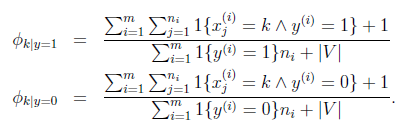事实上，多项式事件模型比之前的模型要好，可能是因为考虑了词出现的次数这个因素。但此问题仍存在争论。

非线性分类算法

例：logistic回归中，假设值小于0.5预测0，大于0.5预测1。即给定一个训练集，logistic回归会找到一条直线（牛顿方法或梯度下降），将正负样本合理分开。但有时数据不能被一条直线分开，需要一种算法，学习非线性的分界线。

上一讲的推论：

x|y=1 ~ ExpFamily(η1)，x|y=0 ~ ExpFamily (η0)  =>  p(y=1|x)是logistic函数

即x|y的分布属于指数分布族，可推出后验分布是logistic函数。

朴素贝叶斯模型也属于指数分布族，所以也是用logistic线性分类器。下面介绍一种非线性分类器。

2、 神经网络

假设特征是x0,x1,x2,x3，x0设置为1，用连线表示logistic回归单元，圆圈表示计算节点，下图中间的节点以x0等特征作为输入，hθ(x)作为输出，这是一个sigmoid函数。为了找到非线性的界限，需要找到一种方式，表示出能够输出非线性分界限的假设。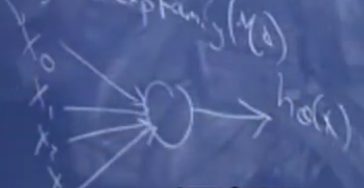将之前画的小单元拼在一起，得到神经网络。特征输入到若干个sigmoid单元，在输入到另外一个sigmoid单元，得到输出。中间节点的输出值设为a1,a2,a3。这些中间节点称为隐藏层，神经网络可以由多个隐层。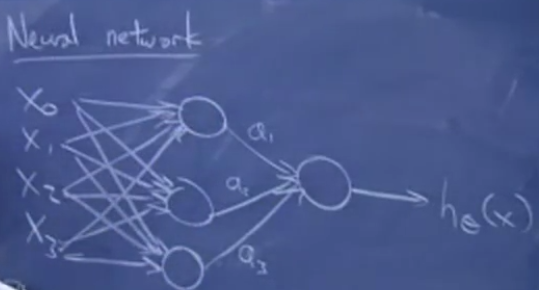每个中间节点有一系列参数：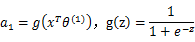a2,a3同理。g为sigmoid函数。最终的输出值为：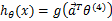其中，a向量由a1,a2,a3组成。

一种学习模型参数的方法是，利用成本函数J(θ)，使用梯度下降使J(θ)最小。即用梯度下降使得神经网络的预测结果和你观察到的训练集中的样本标签尽可能接近。在神经网络中，这种方法称为反向传播。

3、 支撑向量机铺垫 – 最大间隔分类器

另外一种能生成非线性分类器的学习算法。本节课先介绍另外一类线性分类器，在下一讲或者下下讲中，利用支撑向量机的想法，进行一些巧妙的改动和扩展，让它可以生成非线性分界线。

两种对于分类的直观理解：

1)       考虑logistic回归，计算θTx：

输出1  <=> θTx>=0；输出0  <=> θTx<0

如果θTx>>0，相当确定的预测y=1；如果θTx<<0，相当确定的预测y=0

对于所有的i，如果y=1，θTx(i)>>0，如果y=0，θTx(i)<<0，那么我们认为分类器是良好的。即如果我们根据训练集找到了参数，我们的学习算法不仅需要保证分类结果正确，更要进一步保证分类结果的确定性。

2)       假设训练集是线性可分割的，即一定有一条直线可以将训练集分开。那么直观来看，我们一定会选择和正负样本都有一定距离的直线。后面讲到分类器的几何间隔时，再正式讨论。

支撑向量机中改动的符号：

输出y∈{-1,+1}

h输出的假设值也改为{-1,+1}

g(z) = { 1 , 如果z>=0;  -1, 如果z<0}

之前在使用式：hθ(x)=g(θTx)时，假设x0=1且x为n+1维向量，现在忽略这两个假设，表示为：hw.b(x)=g(wTx+b)，这里的b相当于原来的θ0，w相当于原来θ除去θ0剩余部分，长度为n维。将截距b单提出来，方便引出支撑向量机。

函数间隔：

一个超平面(w,b)和某个特定训练样本(x(i),y(i))对应的函数间隔定义为：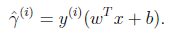参数(w,b)定义了一个分类器，例如定义了一个线性分界线。

如果y(i)=1，为了获得较大的函数间隔，需要令wTx(i)+b >> 0；

如果y(i)=-1，为了获得较大的函数间隔，需要令wTx(i)+b << 0

如果y(i)(wTx(i)+b) > 0，意味着分类结果正确

一个超平面(w,b)和整个训练集的函数间隔定义为：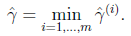即相对于整个训练集的函数间隔定义为所有相对于样本的函数间隔的最坏情形（上述讲到，分界线距离样本越远效果越好）。

几何间隔：

几何距离定义为：一个训练样本对应的点到由超平面确定的分隔线的距离。如下图A到分隔线的距离AB就是几何距离。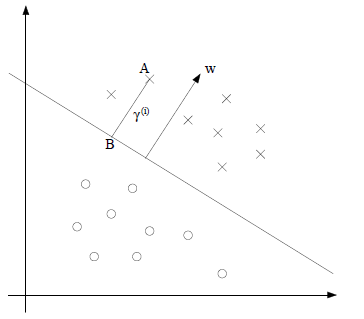和分隔线垂直的单位向量表示为：w/||w||，AB这段距离表示为γ(i)，γ上有小三角表示函数间隔，没有表示几何间隔。若A点表示x(i)，那么点B表示为：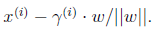由于点B在分隔线上，它应该还满足：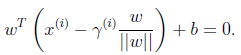可以解出：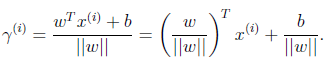上式说明，对于一个训练样本x(i)，到由参数w和b确定的分隔平面之间的距离，可以由上式得到。

由于上述一直假设对样本进行了正确的分类，所以更一般的，将几何间隔定义为：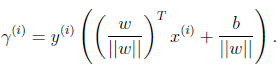这个定义和函数间隔很相似，不同点是对向量w进行了标准化。同样，希望几何间隔也是越大越好。

结论：如果||w||=1，函数间隔等于几何间隔。更一般的，几何间隔等于函数间隔除以||w||。

一个超平面(w,b)和整个训练集的几何间隔定义为：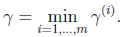和函数间隔类似，取样本中最小的几何间隔。

最大间隔分类器可以看做是支撑向量机的前身，是一种学习算法，选择特定的w和b，使几何间隔最大化。最大分类间隔是下述这样的优化问题：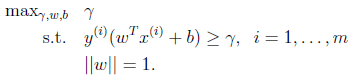即选择γ，w，b是γ最大，同时满足条件：所选取的最大几何间隔必须保证每个样本的结合间隔至少为γ。

最大间隔分类器的效果和logistic回归结果差不多好，深入研究这个分分类器，可以用一种更巧妙的方法让其支持无限维的特征空间，得到有效的非线性分类器。

展开全文• 朴素贝叶斯生成模型，和高斯判别分析类似，主要针对最大化p(X∣Y)p(Y)p(X|Y)p(Y)p(X∣Y)p(Y)进行建模，高斯判别分析有两个假设：对于两类样本，其服从伯努利分布，而对每个类中的样本，假定都服从高斯分布(多属性...
朴素贝叶斯介绍回顾高斯判别分析朴素贝叶斯朴素贝叶斯法模型朴素贝叶斯法的假设朴素贝叶斯法的参数估计极大似然估计贝叶斯估计
回顾
高斯判别分析
生成模型，使用较强的假设。
对于两类样本，其服从伯努利分布，而对每个类中的样本，假定都服从高斯分布。
朴素贝叶斯
朴素贝叶斯是生成模型，和高斯判别分析类似，主要针对最大化$p(X|Y)p(Y)$进行建模，高斯判别分析有两个假设：对于两类样本，其服从伯努利分布，而对每个类中的样本，假定都服从高斯分布(多属性即多维高斯分布）。但是高斯判别分析求解过程非常复杂，所以朴素贝叶斯对数据的属性之间的关系作出了假设，每个类中的样本都服从独立同分布，即iid。
朴素贝叶斯法模型
朴素贝叶斯对数据的属性之间的关系作出了假设，一般地，我们若需要得到 $p(X|Y)$ 这个概率值，由于 $x$ 有 $p$ 个维度，因此需要对这么多的维度的联合概率进行采样，但是我们知道这么高维度的空间中采样需要的样本数量非常大才能获得较为准确的概率近似，但在朴素贝叶斯中采用的是对条件概率分布作出了条件独立性的假设，使得贝叶斯求解大大简化，因此朴素贝叶斯因此得名。
$p(x|y)=\prod\limits_{i=1}^pp(x_i|y)$
即：
$x_i\perp x_j|y,\forall\ i\ne j$
于是利用贝叶斯定理，对于单次观测：
$p(y|x)=\frac{p(x|y)p(y)}{p(x)}=\frac{\prod\limits_{i=1}^pp(x_i|y)p(y)}{p(x)}$
朴素贝叶斯法的假设
对于单个维度的条件概率以及类先验作出进一步的假设：

$x_i$ 为连续变量：$p(x_i|y)=\mathcal{N}(\mu_i,\sigma_i^2)$ ，即一维高斯分布
$x_i$ 为离散变量：类别分布（Categorical）：$p(x_i=i|y)=\theta_i,\sum\limits_{i=1}^K\theta_i=1$
$p(y)=\phi^y(1-\phi)^{1-y}$

对这些参数的估计，常用极大似然估计的方法直接在数据集上估计，由于不需要知道各个维度之间的关系，因此，所需数据量大大减少了。估算完这些参数，再代入贝叶斯定理中得到类别的后验分布。
朴素贝叶斯法的参数估计
估计相应的概率$p(y)$和$p(x_i|y)$
极大似然估计
其实就是根据数据统计一下概率即可

计算$p(y)$

$P(Y=c_k) = \frac{\sum_{i=1}^NI(y_i=c_k)}{N},k=1,2,...,K$

计算$p(x_i|y)$

$P(X^{(j)}=a_{jl}|Y=c_k) = \frac{\sum_{i=1}^NI(x_i^{(j)}=a_{jl},y_i=c_k)}{\sum_{i=1}^NI(y_i=c_k)}$
$j=1,2,...,n;l=1,2,...,S_i;k=1,2,...,K$

给定输入，输出预测标签

给定$x＝(2,S)$，输出$y=-1$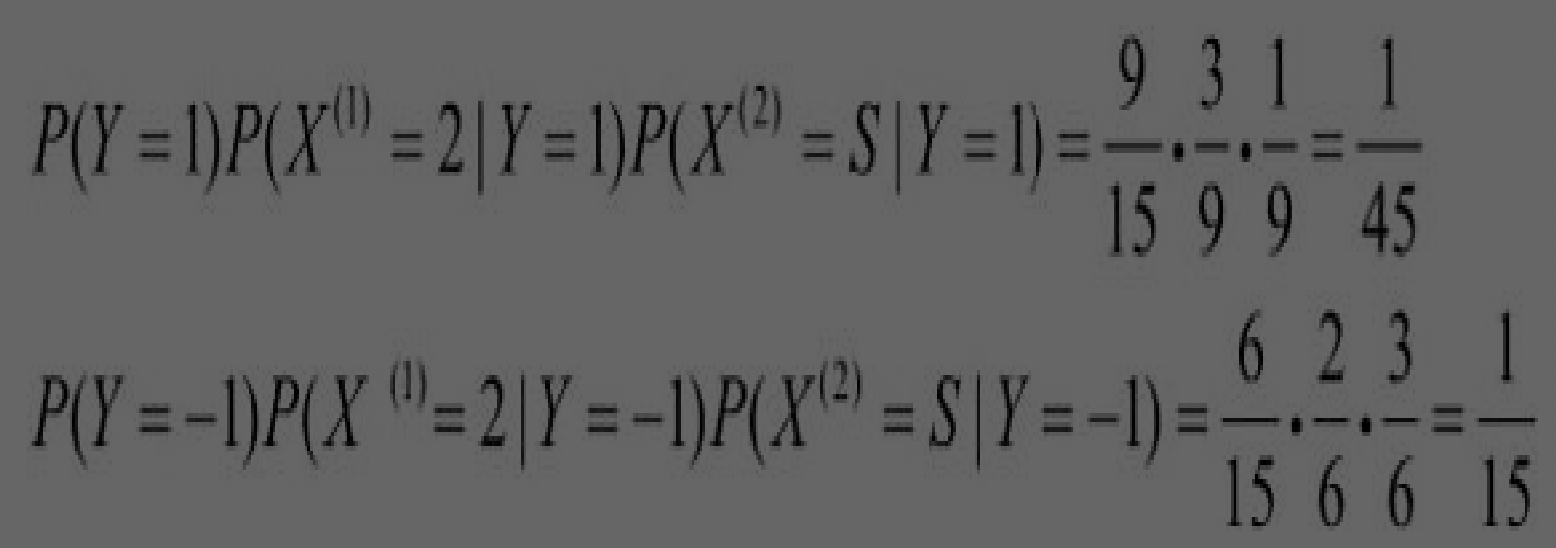贝叶斯估计
可以看到计算概率时用的是连乘，用极大似然估计可能会出现所要估计的概率值为0的情况，所以当有一种特征在此前没出现过，就会导致该标签输出的概率直接为0。因此可使用贝叶斯估计解决这一问题，贝叶斯估计其实非常繁琐，但在该问题的强假设：独立同分布下，就是在取值频数时赋予一个正数对其进行平滑，避免了0值的出现。

计算$p(y)$

$P(Y=c_k) = \frac{\sum_{i=1}^NI(y_i=c_k)+\lambda}{N +K\lambda},k=1,2,...,K$

计算$p(x_i|y)$

$P(X^{(j)}=a_{jl}|Y=c_k) = \frac{\sum_{i=1}^NI(x_i^{(j)}=a_{jl},y_i=c_k)+\lambda}{\sum_{i=1}^NI(y_i=c_k)+S_j\lambda}$
$j=1,2,...,n;l=1,2,...,S_i;k=1,2,...,K$

给定输入，输出预测标签


展开全文• 朴素贝叶斯或者说基于贝叶斯理论的决策方法都是生成模型。...如果先对联合概率分布P(x,c)建模，然后再由此获得P(c|x)，这样得到的生成模型，例如朴素贝叶斯朴素贝叶斯应用的先决条件是“属性条件独...
朴素贝叶斯或者说基于贝叶斯理论的决策方法都是生成式模型。那么什么是生成式模型呢？生成式模型和判别式模型的概念分别是什么？大体来说，给定数据集x，可以直接通过建模P(c|x)来预测c，这样得到的是判别式模型。像BP网络，支持向量机，决策树都属于判别式模型。如果先对联合概率分布P(x,c)建模，然后再由此获得P(c|x)，这样得到的生成式模型，例如朴素贝叶斯。
朴素贝叶斯应用的先决条件是“属性条件独立假设”，即已知类别，假设所有属性相互独立。转载于:https://www.cnblogs.com/LittleRea/p/7554321.html
展开全文• 一、全概率公式与贝叶斯公式 下面是是全概率公式和...朴素贝叶斯算法属于生成模型，需要对p(x|y)进行建模，如下图所示： p(y)称为先验概率，他给出的是属于那一个类别的概率，这个一般比较好计算。p(x|y)是lik...贝叶斯公式 贝叶斯估计
• 文章目录判别模型与生成模型判别模型生成模型先验概率、条件概率、后验概率朴素贝叶斯建模后验概率P(Y=ck∣X=x)P(Y=c_k| X = x)P(Y=ck​∣X=x)最大化的解释朴素贝叶斯法的参数估计极大似然估计算法流程贝叶斯估计...机器学习 人工智能 算法 数据挖掘
• 另外，它是一种生成模型（generative model），采用直接对联合概率P(x,c)建模，以获得目标概率值的方法。预备知识 先验概率与后验概率 贝叶斯定理Bayesian Theorem 朴素贝叶斯分类器 何为朴素属性条件独立性假设 ...
• 朴素贝叶斯采取了属性条件...生成模型：先对联合概率分布建模，然后由此获得 对贝叶斯定理可写为： 贝叶斯学派认为参数是未观察到的随机变量，其本身也有分布，因此可以假设参数服从先验分布，然后基于观察到的数...
• 贝叶斯定理已知某条件概率，如何得到两个时间交换后的概率，也就是在已知P(A|B)的情况下如何求得P(B|A) 朴素贝叶斯分类朴素贝叶斯（分类器）是一种生成模型，它会基于训练样本对每个可能的类别建模。之所以叫朴素...
• 在机器学习中，生成模型可以用来直接对数据建模（例如根据某个变量的概率密度函数进行数据采样），也可以用来建立变量间的条件概率分布。条件概率分布可以由生成模型根据贝叶斯定理形成。常见的基于生成模型算法有...
• 朴素贝叶斯属于监督学习的生成模型，实现简单，没有迭代，学习效率高，在大样本量下会有较好表现。但因为假设太强——特征条件独立，在输入向量的特征条件有关联的场景下，并不适用。 朴素贝叶斯算法：主要思路是...
• -朴素贝叶斯事件模型 2、神经网络（简要） 3、支撑向量机（SVM）铺垫–最大间隔分类器 复习： 1、朴素贝叶斯 一种生成学习算法，对p(x|y)建模。 例：垃圾邮件分类 以邮件输入流作为输入，输出y为{0,1}，1...
• 本节为吴恩达教授机器学习课程第四部分，生成学习算法（2），包括：朴素贝叶斯算法，拉普拉斯平滑，并在结尾附上一个中文文本分类系统的实现代码链接。 2. 朴素贝叶斯   GDA中特征向量时连续的实值向量，朴素...机器学习
• Content 相关概念生成模型判别模型先验概率，后验概率与条件概率贝叶斯决策理论下溢问题如何解决 相关概念 生成模型 生成模型：在概率统计理论中, 生成模型是指能够随机生成观测数据的模型，尤其是在给定某些...机器学习
• 在机器学习中，生成模型可以用来直接对数据建模（例如根据某个变量的概率密度函数进行数据采样），也可以用来建立变量间的条件概率分布。条件概率分布可以由生成模型根据贝叶斯定理形成。常见的基于生成模型算法有...python 机器学习
• 在机器学习中，生成模型可以用来直接对数据建模（例如根据某个变量的概率密度函数进行数据采样），也可以用来建立变量间的条件概率分布。条件概率分布可以由生成模型根据贝叶斯定理形成。常见的基于生成模型算法有...
• 朴素贝叶斯事件模型 2、 神经网络（简要） 3、 支撑向量机（SVM）铺垫 – 最大间隔分类器   复习： 1、 朴素贝叶斯 一种生成学习算法，对p(x|y)建模。   例：垃圾邮件分类 以邮件输入流作为输入...机器学习 计算机视觉 神经网络
• 首先，我们举个例子来说明什么是生成学习算法：  假设要判定一个病人所患的是癌症是...生成学习算法概念：对两个类别的样本分别进行建模，用新的样本去匹配两个模型，匹配度较高的作为新样本的类别。 判别算法的概高斯判别
• 朴素贝叶斯和高斯判别分析都是生成模型，并且都针对联合概率进行建模： y=argmaxy∈{0,1}p(y∣x)=argmaxy∈{0,1}p(y)p(x∣y)y=argmax_{y\in\left\{0,1\right\}}p(y|x)=argmax_{y\in\left\{0,1\right\}}p(y)p(x|y)y...
• 贝叶斯分类器学习笔记贝叶斯决策论估计似然和先验概率朴素贝叶斯分类器 贝叶斯决策论 对于一些细节的想法（如有错误以后会更正） 其中，将x分为所有类的概率和为1，因此才有了 时，， 即1减去分类正确的条件概率。...机器学习
• 在机器学习中，生成模型可以用来直接对数据建模（例如根据某个变量的概率密度函数进行数据采样），也可以用来建立变量间的条件概率分布。条件概率分布可以由生成模型根据贝叶斯定理形成。常见的基于生成模型算法有...机器学习
• 1、关于生成模型和判别模型，我一开始也很迷糊，后来我发现只要记住一点：生成模型首先是概率模型，要计算后验概率，但不是直接计算后验概率，得先通过计算联合概率，然后比较联合概率的大小，间接比较（得到）后验...机器学习
• 一、判别式学习算法和生成式学习算法 ...生成学习算法则是对两个类别分别进行建模，用新的样例去匹配两个模型，匹配度较高的作为新样例的类别。 二、贝叶斯公式 朴素贝叶斯算法的核心公式自然是贝...
• 之前讲的回归模型属于判别模型，今天引入新的模型——生成学习算法：对训练集建立两个概率模型，测试特征代入两个模型比较哪个模型的最终概率高来判断类别（而不是计算出两个概率，而是比较两个概率） 利用贝叶斯...高斯判别分析 Laplace平滑 logistic回归
• 像逻辑回归，用hθ(x) = g(θTx) 直接地来建模p(y|x; θ) ；或者像感知机，直接从输入空间映射到输出空间（0或1），它们都被称作判别学习（discriminative learning）。与之相对的是生成学习（generative learning）...
• 在正式学习朴素贝叶斯之前，需要明确的是机器学习所要实现的是基于有限的训练样本集尽可能准确地估计出后验概率P(c|x)，即根据特征得到所属类别的概率，首先引入两个概念。判别式模型（discriminative models）：...
• 生成模型（Generative model） 判别模型（Discriminative model） 对特征x和目标y的联合分布P(x,y)建模，使用极大后验概率估计法估计出最有可能的P(y|x) 直接对后验概率P(y|x)建模，使用极大似然...
• （一）生成学习算法 在线性回归和Logistic回归这种类型的学习算法中我们探讨的模型都是p(y|x;θ)，即给定x的情况探讨y的条件概率分布。如二分类问题，不管是感知...而生成学习算法则是对两个类别分别进行建模，用新...